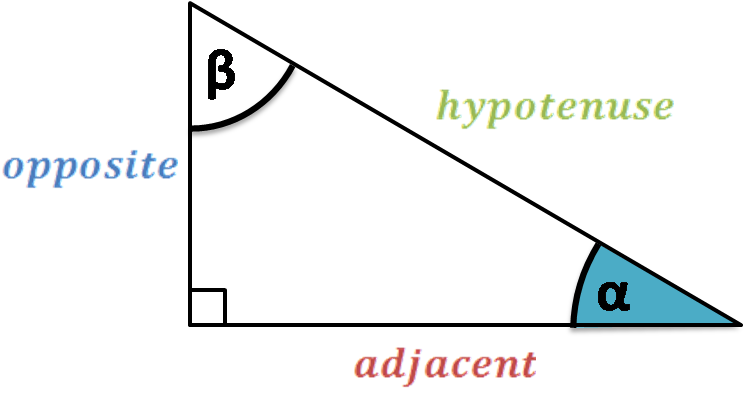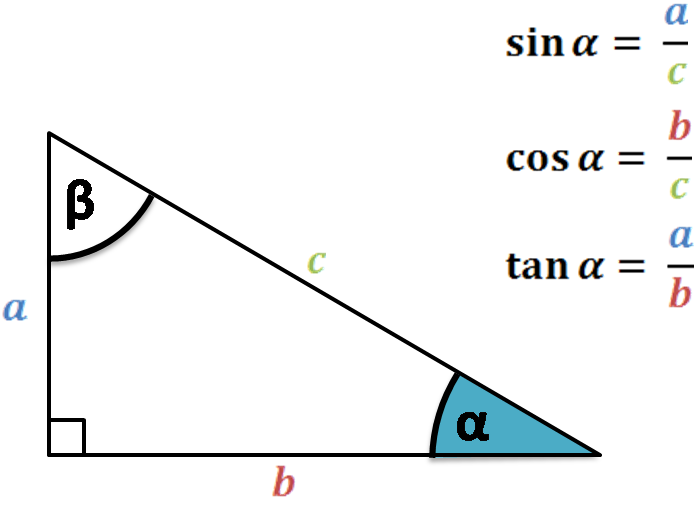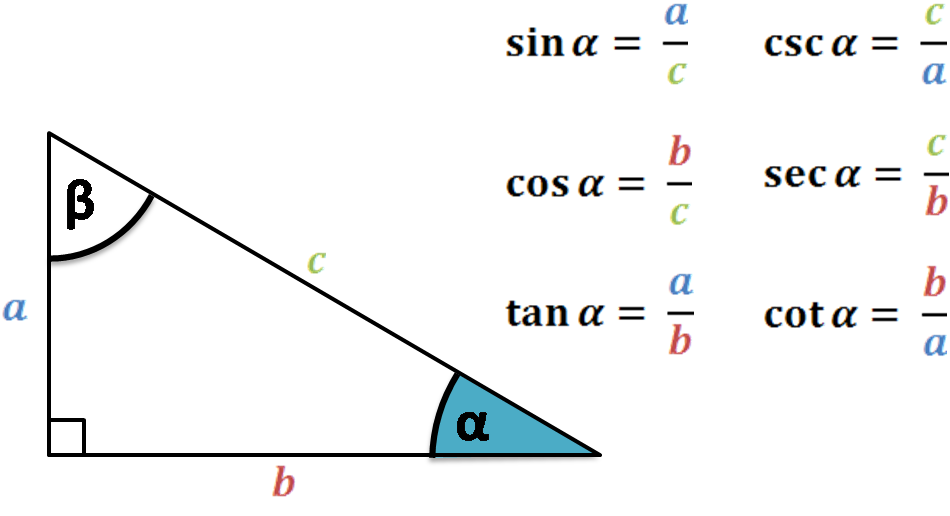# Trigonometric Functions Calculator

Created by Luis Hoyos
Last updated: Jul 05, 2022

Welcome to our trigonometric functions calculator, where you'll be able to find the six trigonometric functions values.

Here, you'll find the three trigonometric functions (aka circular functions) - sine, cosine, and tangent, but also their reciprocals: cosecant, secant, and cotangent, respectively.

Keep reading to learn more about trigonometric ratios used to calculate the circular functions and how to use this trigonometric functions calculator. If you're familiar with basic trigonometric functions, then check our other tool to learn what arcsin is!

## Trigonometric ratios

Sine, cosine, and tangent are the most common circular functions of an angle. For acute angles (those which measure less than 90°), you can define these functions in the context of a right triangle, whose sides are:• Hypotenuse - you probably heard that term when learning about the Pythagorean theorem. The hypotenuse is the opposite side to the right angle (90°) and is the triangle's longest side.
• Opposite side - this is the side opposite the angle of interest (in this case, angle α).
• Adjacent side - this is the remaining side, which is not the hypotenuse nor the side opposite the angle of interest.

Now that we know the sides terminology, we can define the trigonometric functions:

• sin(α) = opposite/hypotenusesin(α) = a/c
• cos(α) = adjacent/hypotenusecos(α) = b/c
• tan(α) = opposite/adjacenttan(α) = a/bCosecant, secant, and cotangent are other commonly calculated circular functions:

• csc(α) = 1 / sin(α) → c / a
• sec(α) = 1 / cos(α) → c / b
• cot(α) = 1 / tan(α) → b / a

The csc(α) and sec(α) have the same range, which is y ≥ 1 and y ≤ -1, while the range cot is all real numbers.Because we express them as ratios of different sides, trigonometric functions are also known as trigonometric ratios.

## How to find the six trigonometric functions using this calculator

To use this trigonometric functions calculator, just follow these two simple steps:

1. Input the angle. You can choose between degrees, radians, or π rad. For example, if your angle is π/2, you must select the π rad unit and type 1/2.
2. That's it! That's how you find the six trigonometric functions with this trigonometric functions calculator.
Luis Hoyos
Angle
deg
People also viewed…